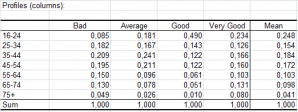# Correspondence Analysis (CA)

Correspondence analysis is a statistical method used to investigate the relationship between two qualitative variables. Do it in Excel with the XLSTAT software.## What is Correspondence Analysis

Correspondence Analysis is a powerful method that allows studying the association between two qualitative variables. It is based on the measure of the inertia.

The aim of Correspondence Analysis is to represent as much of the inertia on the first principal axis as possible, a maximum of the residual inertia on the second principal axis and so on until all the total inertia is represented in the space of the principal axes. One can show that the number of dimensions of the space is equal to min(m1, m2)-1.

Four approaches of the Correspondence Analysis are proposed by XLSTAT:

• Classical Correspondence Analysis (CA)

• Non-Symmetrical Correspondence Analysis (NSCA)

• Correspondence Analysis using the Hellinger distance (HD)

• Detrended Correspondence Analysis (DCA)

## Options for Correspondence Analysis in XLSTAT

### Non-Symmetrical Correspondence Analysis

Non-Symmetrical Correspondence Analysis (NSCA), developed by Lauro and D’Ambra in 1984, analyzes the association between the rows and columns of a contingency table while introducing the notion of dependency between the rows and the columns, which leads to an asymmetry in their treatment.

The XLSTAT algorithm allows computing both CA and the related method of NSCA in a similar way.

The Advanced analysis option in the dialog box allows you to choose the type of analysis you want to perform on the data. The analysis on supplementary data and the analysis of a subset are only active if the selected data correspond to a contingency table or a more general two-way table. The possible options are:

• Supplementary data: If you select this option you may then enter the number of supplementary rows and/or columns. Supplementary rows and columns are passive data that are not taken into account for the computation of the representation space. Their coordinates are computed a posteriori. Notice that supplementary data should be the last rows and/or columns of the data table.

• Subset analysis: If you select this option you can then enter the number of rows and/or columns to exclude from the subset analysis. Notice that the excluded data should be the last rows and/or columns of the data table.

• Detrended Correspondence Analysis: If you select this option you may then enter the parameters that are useful for the calculations, i.e. the number of segments to cut the axes and the number of rescalings to perform. By default, the number of segments is set to 26 and the number of rescalings is set to 4.

The Detrended Correspondence Analysis (DCA) is a method proposed by Hill and Gauch (1980), mainly used on ecological data. The aim of this method is to correct drawbacks (e.g. "arc effect") encountered when using classical CA.

### Distance measures in Correspondence Analysis

The Distance option in the dialog box lets you compute a Correspondence Analysis based on the Chi-square distance or on the Hellinger distance as proposed by Rao (1995).

• Chi-Square: Select this option to compute classical Correspondence Analysis.

• Hellinger: Select this option to compute Correspondence Analysis based on Hellinger distance (HD). This option is not available if the "Non-symmetrical analysis" option has been selected or the case of the Detrended Correspondence Analysis.### analyze your data with xlstat

14-day free trial

Included in

Related features